# CW structure of real projective space

This article discusses a common choice of CW structure for real projective space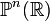$\mathbb{P}^n(\R)$, i.e., a CW-complex having this as its underlying topological space.

## Description of cells and attaching maps

There is one cell in dimension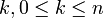$k, 0 \le k \le n$. Thus, there is a total of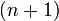$(n + 1)$ different cells. Note that:

• The$k$-skeleton is homeomorphic to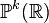$\mathbb{P}^k(\mathbb{R})$, and in fact, the CW structure induced on this skeleton is the same as the CW structure we would have chosen for$\mathbb{P}^k(\mathbb{R})$ independently
• The attaching map at stage$k + 1$ is the map arising from the fiber bundle of sphere over projective space (complex case)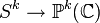$S^k \to \mathbb{P}^k(\mathbb{C})$.

A more concrete way of interpreting these cells and attaching maps is as follows. Choose a basis for$\mathbb{R}^{n+1}$.$\mathbb{P}^n(\mathbb{R})$ is the space of lines through the origin in$\mathbb{R}^{n+1}$. The$k$-skeleton is the subspace comprising those lines that lie inside the subspace spanned by the first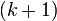$(k + 1)$ basis vectors. Each time we add a new cell, we are allowing directions that lie in the span of one more basis vector.

## Cellular chain complex and cellular homology

Any CW structure on a topological space provides a cellular filtration relative to the empty space. The corresponding cellular chain complex is described below. By excision, the$k^{th}$ cellular chain group is$\mathbb{Z}^d$ where$d$ is the number of$k$-cells.

For the case of$\mathbb{P}^n(\mathbb{C})$, since there is one cell in dimension$2k$ for$0 \le k \le n$, the cellular chain groups are$\mathbb{Z}$ in dimensions$2k$ for$0 \le k \le n$ are$0$ elsewhere.

The cellular chain complex thus looks like:$\dots \to 0 \to 0 \to 0 \to \mathbb{Z} \to \mathbb{Z} \to \mathbb{Z} \to\dots \to \mathbb{Z} \to \mathbb{Z} \to \mathbb{Z}$

The boundary maps are as follows: the map$H_k \to H_{k-1}$ for$k$ even,$k \le n$ is the doubling map. For$k$ odd, the map is the zero map.

This gives the homology groups as described in homology of real projective space.